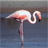# New to Qlik Sense

If you’re new to Qlik Sense, start with this Discussion Board and get up-to-speed quickly.

cancel
Showing results for
Did you mean:Contributor II

## Weighted medians - Running totals

Hi,

I want to find the weighted median for a range of weighted values. I have all of the components but unsure how to combine them in order to get the value I needI have  response_% and then the weighting factor that corresponds to each response. I then have a running total of the weighting factor and then a column showing the sum of the weighting factor / 2.

I need to return the response_% value where the running total equals 50% (527.7th) which in this case is 2286960

I was thinking along the lines of:

only {<Rangesum(Above(TOTAL Sum([Weighting_factor_%]),0,rowno(TOTAL)))  = sum([Weighting_factor_%]) / 2 >}(Response_%)

but obviously the set analysis doesn't work

Any ideas?

Sophie

Labels (5)

• ### Weighted median

0 RepliesTags
Community Browser